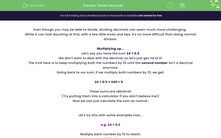# Divide Decimals

In this worksheet, students will practise dividing whole numbers and decimals by each other, removing the decimal places by multiplying both numbers by multiples of 10.Key stage:  KS 4

Year:  GCSE

GCSE Subjects:   Maths

GCSE Boards:   Pearson Edexcel, OCR, Eduqas, AQA,

Curriculum topic:   Number, Fractions, Decimals and Percentages

Curriculum subtopic:   Structure and Calculation Decimal Fractions

Popular topics:   Decimals worksheets

Difficulty level:#### Worksheet Overview

Even though you may be able to divide, dividing decimals can seem much more challenging.

While it can look daunting at first, with a few little tricks and tips, it's no more difficult than doing normal division.

Multiplying up...

Let's say you have the sum 24 ÷ 0.5

We don't want to deal with the decimal, so let's just get rid of it!

The trick here is to keep multiplying both the numbers by 10 until the second number isn't a decimal anymore.

Going back to our sum, if we multiply both numbers by 10, we get:

24 ÷ 0.5 = 240 ÷ 5

These sums are identical!

(Try putting them into a calculator if you don't believe me!)

Now we can just calculate the sum as normal.

Let's try this with some examples now...

e.g. 24 ÷ 0.2

Multiply each number by 10 to reach:

240 ÷ 2

Then calculate the sum as normal:

24 ÷ 0.2 = 120

It's as simple as that!

e.g. 375 ÷ 0.05

Multiply each number by 10 to reach:

3750 ÷ 0.5

As the second number is still a decimal, we need to multiply by 10 again to get:

37500 ÷ 5

Now we can calculate as normal!

375 ÷ 0.05 = 7500

In this activity, you will practise dividing whole numbers and decimals by each other, removing the decimal places by multiplying both numbers by multiples of 10.

### What is EdPlace?

We're your National Curriculum aligned online education content provider helping each child succeed in English, maths and science from year 1 to GCSE. With an EdPlace account you’ll be able to track and measure progress, helping each child achieve their best. We build confidence and attainment by personalising each child’s learning at a level that suits them.

Get started